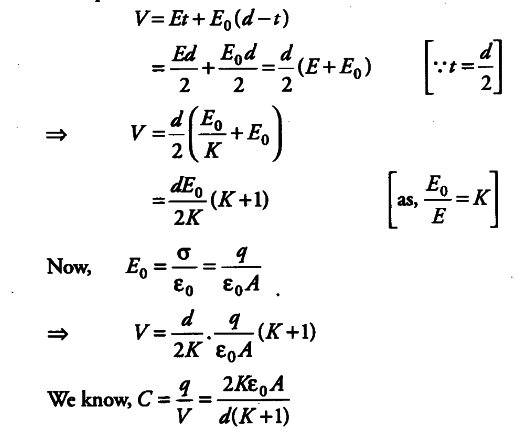# A slab of material of dielectric constant K has the same area as that of the plates

A slab of material of dielectric constant K has the same area as that of the plates of a parallel plate capacitor but has the thickness d/2, where d is the separation between the plates. Find out the expression for its capacitance, when the slab is inserted between the plates of the capacitor

Suppose that the capacitor is connected to a battery, an electric field { E }_{ 0 } is produced. Now, if we insert the dielectric slab of thickness t = d/ 2, the electric field reduces to E.
Now, the gap between plates is divided in two parts, for distance t there is electric field E and for the remaining distance (d—t) the electric field is { E }_{ 0 }
If V be the potential difference between the plates of the capacitor, then